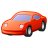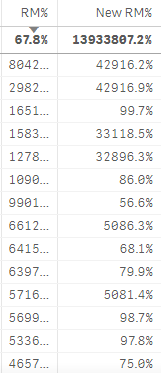# New to Qlik Sense

If you’re new to Qlik Sense, start with this Discussion Board and get up-to-speed quickly.

Announcements
Welcome to Qlik Community! Check out our new navigation! FIND OUT MORE
cancel
Showing results for
Did you mean:Partner - Contributor III

## Getting Values More than equal to Total

Hi Experts,

I have calculated RM% . Now my requirement is based on selection the records which has the value greater than equal to total RM% on visible. For Example

In below image my Avg. RM% is 69.6% and now I want only those records in my table whose RM% Value is greater than equal to  69.6%.

Like from the image 82.6%,71.9%,339.5% and all.

Labels (4)

• ### greater than in measure

15 RepliesPartner - Contributor III
Author

As per below sample I got the total RM% 74.0%, Now I need only those records in the table which has the RM% values greater than equal to Total RM% Value i.e more than equal to 74.0%.

like 87.2%, 91.5%,374.1%,103.9% etc.

I think this will give you the clear picture.

 PlantCode Material No Fiscal Year Fiscal Month BOM Base Price [Billing Qty] RM% Total - - - - - - 74.0% BP0X BP02100000133243 2018-2019 Sep 6.69 1083.6 120 0.0% BP0X BP02100000136273 2018-2019 Sep 0.2 16832.8 4240 5.0% BP0X BP02100000212102 2018-2019 Sep 28.45 8807.4 270 87.2% BP0X BP02100000212103 2018-2019 Sep 47.26 13426.4 260 91.5% BP0X BP02100000213102 2018-2019 Sep 28.45 8807.4 270 87.2% BP0X BP02100000213103 2018-2019 Sep 47.26 13426.4 260 91.5% BP0X BP02100000256056 2018-2019 Sep 0.13 1160 2000 22.4% BP0X BP02100000256060 2018-2019 Sep 0.39 4332 1200 10.8% BP0X BP02100000256061 2018-2019 Sep 0.52 801.6 240 15.6% BP0X BP02100000256110 2018-2019 Sep 1.67 3036 1200 66.0% BP0X BP02100000257013 2018-2019 Sep 4.78 4370 950 0.0% BP0X BP02100000257013 2018-2019 Sep 4.78 3450 750 374.1% BP0X BP02100000257013 2018-2019 Sep 4.78 2300 500 0.0% BP0X BP02100000257014 2018-2019 Sep 4.78 4025 875 103.9% BP0X BP02100000257033 2018-2019 Sep 7.1 5151.2 940 129.6% BP0X BP02100000257035 2018-2019 Sep 3.23 6330 1000 98.7%Master II

Yeah ..

1: what total function are you using ?

if you change rm% code to below does the output you are seeing now change ?

```(

Sum({<
[Fiscal Year] = {">=\$(=Max([Fiscal Year]))"}
,Procurement = {'E'}
,[Fiscal Month] //= {"\$(=Month(Max(CalendarDate)))"}
,[Fiscal Quarter]
>}[Billing Qty])
*
Sum({<
[Fiscal Year] = {">=\$(=Max([Fiscal Year]))"}
,[Fiscal Month] //= {"\$(=Month(Max(CalendarDate)))"}
,[Costing Variant] = {'YPC1'}
,[Fiscal Quarter]
>}BOM ))

/
Sum({<
[Fiscal Year] = {">=\$(=Max([Fiscal Year]))"}
,Procurement = {'E'}
//,[Fiscal Month] //= {"\$(=Month(Max(CalendarDate)))"}
,[Fiscal Quarter]
>}[Base Price])

```
Learning never stops.Partner - Contributor III
Author
Yes, Its changed.Master II
An image for that plesase. You didn't answer what total function are you using ?
Learning never stops.Partner - Contributor III
AuthorThis is the new output  I am getting using the logic without aggr function.

No total function I used for calculation RM%, The logic I mentioned before is the only I used for calculating RM%.MVP

@DS14 wrote:

Hi Sunny it's not working for me,

I am calculating RM% using the below expression.

(

sum(
aggr(Sum({<
[Fiscal Year] = {">=\$(=Max([Fiscal Year]))"}
,Procurement = {'E'}
,[Fiscal Month] //= {"\$(=Month(Max(CalendarDate)))"}
,[Fiscal Quarter]
>}[Billing Qty]),PlantCode,[Material No],[Fiscal Month])
*
aggr(Sum({<
[Fiscal Year] = {">=\$(=Max([Fiscal Year]))"}
,[Fiscal Month] //= {"\$(=Month(Max(CalendarDate)))"}
,[Costing Variant] = {'YPC1'}
,[Fiscal Quarter]
>}BOM),PlantCode,[Material No],[Fiscal Month])
)
/
Sum({<
[Fiscal Year] = {">=\$(=Max([Fiscal Year]))"}
,Procurement = {'E'}
//,[Fiscal Month] //= {"\$(=Month(Max(CalendarDate)))"}
,[Fiscal Quarter]
>}[Base Price])

)

If i am using this expression in the way you suggested its not working.

Please suggest something on this.